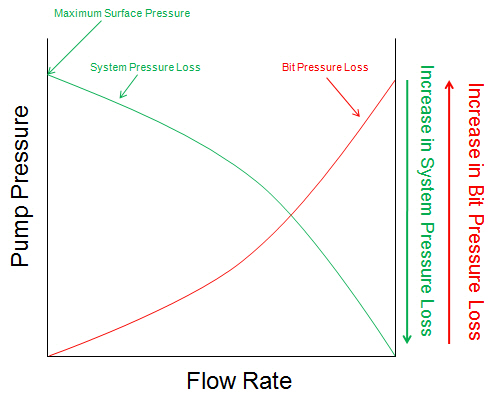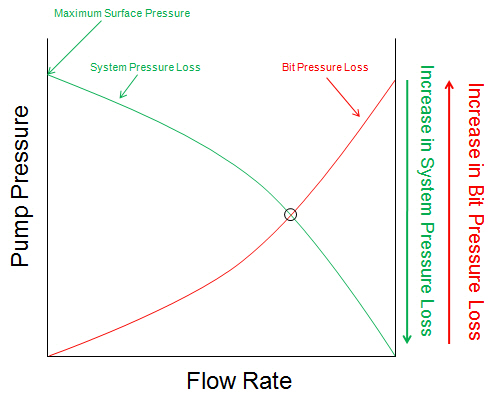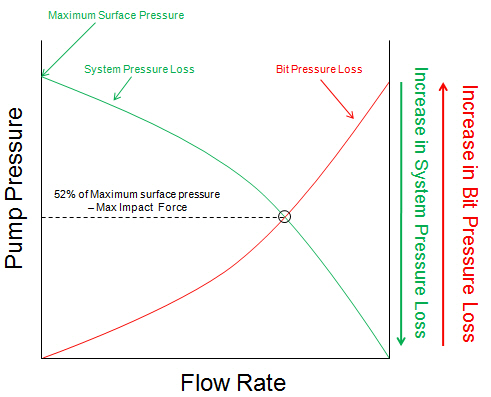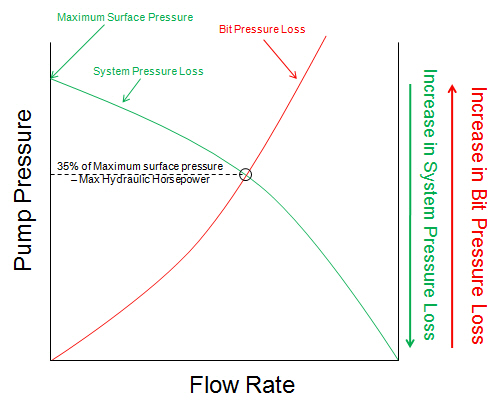# Determine The Optimized Points for Drilling Hydraulics

The design of hydraulic optimization begins with downhole component and an analysis of pressure losses as a function of flow rate.

Before I will explain you about the pressure and flow rate curve, I would like to get the following term across. The surface pressure (pump pressure) is equal to the system pressure loss and the pressure loss across the bit.

P surface = P system + P bit

The curve below demonstrates a system pressure loss curve and a pressure loss across the bit as Y-axis and a flow rate as X-axis. The system pressure loss curve starts at the maximum surface pressure and is drawn invert with the bit pressure loss curve (see the curve below more understanding).The point where two curves cross each other is happened when the summation of system pressure loss and the pressure loss across the bit is equal to maximum surface pressure (see the curve below).What will be happened if you adjust the nozzle size?

Larger nozzle size will move the intersection point to higher flow rate and total pressure loss. Conversely, the smaller nozzle size will move the intersection point backward to lower flow rate and total pressure loss. See the curve below for more understanding.As we’ve mentioned in the previous about two ways of hydraulic optimizations which are maximum impact force and maximum hydraulic horsepower.

Maximum impact force is happened when 48% of maximum surface pressure is bit pressure loss and maximum hydraulic horsepower is happened when 65% of maximum surface pressure is bit pressure loss.

In order to find the optimum point, the easiest way is to determine the flow rate which will give you either maximum impact force or maximum hydraulic horsepower.

For the maximum impact force, you need to draw a constant line at 52% of the maximum surface pressure and determine the intersection point with the system pressure loss curve (see the curve below).For the maximum hydraulic horsepower, you need to draw a constant line at 35% of the maximum surface pressure and determine the intersection point with the system pressure loss curve (see the curve below).When you already find the intersection point which is met your hydraulic optimization criteria, the last task is to figure out the total flow areas (TFA) which will make the bit pressure loss curve pass to the required point. For the detailed calculation, it will be discussed in the later articles.

Reference:Drilling Hydraulic Books

Share the joy